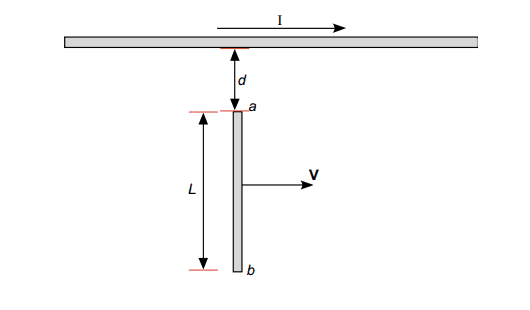# Induced EMF, bar moving through magnetic field

## Homework Statement

A long, straight wire as shown below carries a constant current I. A metal bar with length L is moving at a constant velocity V as shown. Point ‘a’ is a distance ‘d’ from the wire. a) Derive an expression for the EMF induced in the bar. b) Which point ‘a’ or ‘b’ is at a higher potential?## Homework Equations

B=μI/2πr for a straight wire. EMF=Blv for motional EMF.

## The Attempt at a Solution

For part a) I split up the bar into a bunch of small segments of length dr, a distance "r" away from the wire. so dB=μIdr/2πr. Integrating that from point (a) to point (a+b) I get B=(μI/2π)ln((a+b)/a). While I feel reasonably confident about that part, I thought about it a bit more, and I am not sure how multiplying the B-field, μI/2πr by the length, dr, really makes sense, am I forgetting something important? But assuming that is right, I just plug that into EMF=Blv, and get my answer.

Part b) I feel stuck on, only thing I could thing of was using the equation B=μI/2πr and plugging in and (a) and (a+b) for r. Since if I plug in (a) it will be a greater value, then the EMF will be greater there.

rude man
Homework Helper
Gold Member
The B field is not segmented into small sections dB. B is not a differential here.
Do you know the Blv law? It's the expression for emf of a bar of length l moving with velocity v perpendicularly to a B field such that B, l and v are all orthogonal to each other. Now, what is the right expression for a differential element of emf based on this law?

The B field is not segmented into small sections dB. B is not a differential here.
Do you know the Blv law? It's the expression for emf of a bar of length l moving with velocity v perpendicularly to a B field such that B, l and v are all orthogonal to each other. Now, what is the right expression for a differential element of emf based on this law?
I worked on it some more and got d(EMF)=B(r)vdr which simplifies to d(EMF)=(μI/2πr)vdr, with limits for the right side of "a" to "a+b". Also B(r) is the B-field a distance "r" away from the wire.
Forgot to add that for part b) I used the right hand rule and got the the magnetic force, Fb is pointing towards point a for a positive charge inside the rod, so the positive charges will go towards point a and the negative charges towards point b, so point a is at a higher potential.

rude man
Homework Helper
Gold Member
I worked on it some more and got d(EMF)=B(r)vdr which simplifies to d(EMF)=(μI/2πr)vdr, with limits for the right side of "a" to "a+b". Also B(r) is the B-field a distance "r" away from the wire.
Forgot to add that for part b) I used the right hand rule and got the the magnetic force, Fb is pointing towards point a for a positive charge inside the rod, so the positive charges will go towards point a and the negative charges towards point b, so point a is at a higher potential.
That looks 100% right.

That looks 100% right.
Great, thanks!